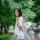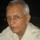Brainy Puzzles
Puzzle Solvers: Interesting brainy puzzles shared by fellow puzzlers
215 Members
Join this group to post and comment.Rashmi Gandhi • Jun 8, 2019

If 8+4 = 212, 9+3 = 312, 4+2 = 26, 8+2 = 410 then what is 5+1 = ?

This was a puzzle from a Kenyan bank. Can you find the correct answer? Do support your answers with explanation of how you came up with it..

WILL post more soon!a+b=(a/b)(a+b)

8+4=(8/4)(8+4)=212

9+3=(9/3)(9+3)=312  and so on.

So, 5+1=(5/1)(5+1)=56Kaustubh Katdare • Jun 8, 2019

Impressive. I've always sucked at solving such problems. 😐@Kaustubh Katdare , you’re too modest. I have seen you cracking some tough nuts in a snap of fingers.Rashmi Gandhi • Jun 13, 2019

Wow! Looks like that's the correct solution. 👍